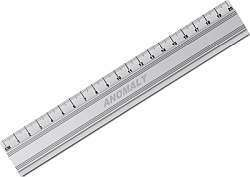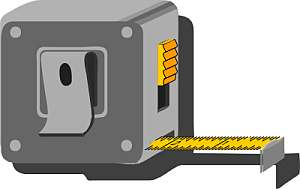# Centimeters to Feet and Inches Calculator

Instructions: Use this calculator to convert a centimeters to feet and inches notation, showing all the steps. Please type the number of centimeters you would like to convert in the form below:Type the number of centimeters:

As you probably have seen from other metric calculators in this site, there are the two main ways of expressing lengths, which are the metric system (using meters and centimeters) and the imperial system (using feet and inches).

The idea for these calculator is to apply conversion formulas, for example, to convert heights in the metric system, let's say something like 173 cms into feet and inches## How do you convert centimeters to feet and inches notation?

Perhaps this is one of the conversion formulas that could pose some complications, though it is still pretty simple.

### Steps for the conversion of centimeters to Feet and Inches

1. Observe that 1 foot is the same as 30.48 centimeters. This will be our starting point
2. Assume you have given C centimeters. You first want to know many feet F are EXACTLY equivalent to those C centimeters
3. The direct proportion that needs to be used is $$\displaystyle \frac{1}{30.48} = \frac{F}{C}$$
4. Solving the proportion for F, we get the following conversion formula: $$F = \displaystyle \frac{C}{30.48}$$
5. We define F* as the floor (integer part) of F (for example, if F = 3.86, then F* = 3). This is the number of feet that will go in the imperial notation
6. We find the number of inches as $$I = \displaystyle\frac{C}{2.54} - 12 F*$$

So as we can see, in this case there is not a conversion formula, but rather a multi-step process, where first the integer number of feet is found, and then the leftover is converted to inches.### Examples: Converting centimeters to feet

What is 173 cms in feet and inches?

Solution: First, using the conversion formula:

$F = \displaystyle \frac{C}{30.48}$

We need to plug the value of C = 173 cms in the above formula, so we get:

$F = \displaystyle \frac{C}{30.48} = \displaystyle \frac{173}{30.48} = 5.676 \text{ feet}$

Taking the floor (the integer part) of F, we get F* = 5. The number of inches is computed as follows

$I = \displaystyle \frac{C}{2.54} + 12 F* = \displaystyle \frac{173}{2.54} - 12 \times 5 = 8.11 \approx 8$

So the conversion is L = 173 cm = 5' 8"

### Another conversion example

Convert 190 cms into feet and inches

Solution: First, using the conversion formula:

$F = \displaystyle \frac{C}{30.48}$

We need to plug the value of C = 173 cms in the above formula, so we get:

$F = \displaystyle \frac{C}{30.48} = \displaystyle \frac{173}{30.48} = 5.676 \text{ feet}$

Taking the floor (the integer part) of F, we get F* = 5. The number of inches is computed as follows

$I = \displaystyle \frac{C}{2.54} + 12 F* = \displaystyle \frac{173}{2.54} - 12 \times 5 = 8.11 \approx 8$

So the conversion is L = 173 cm = 5' 8" approximately

## Other Conversion calculators of interest

You may be interested in some of our other metric conversion calculators, such as our meters to inches calculator, or the centimeters to inches, meters to feet, imperial to centimeters or imperial to meters calculators. All these calculators will have a use, depending on the units you are interested in converting.